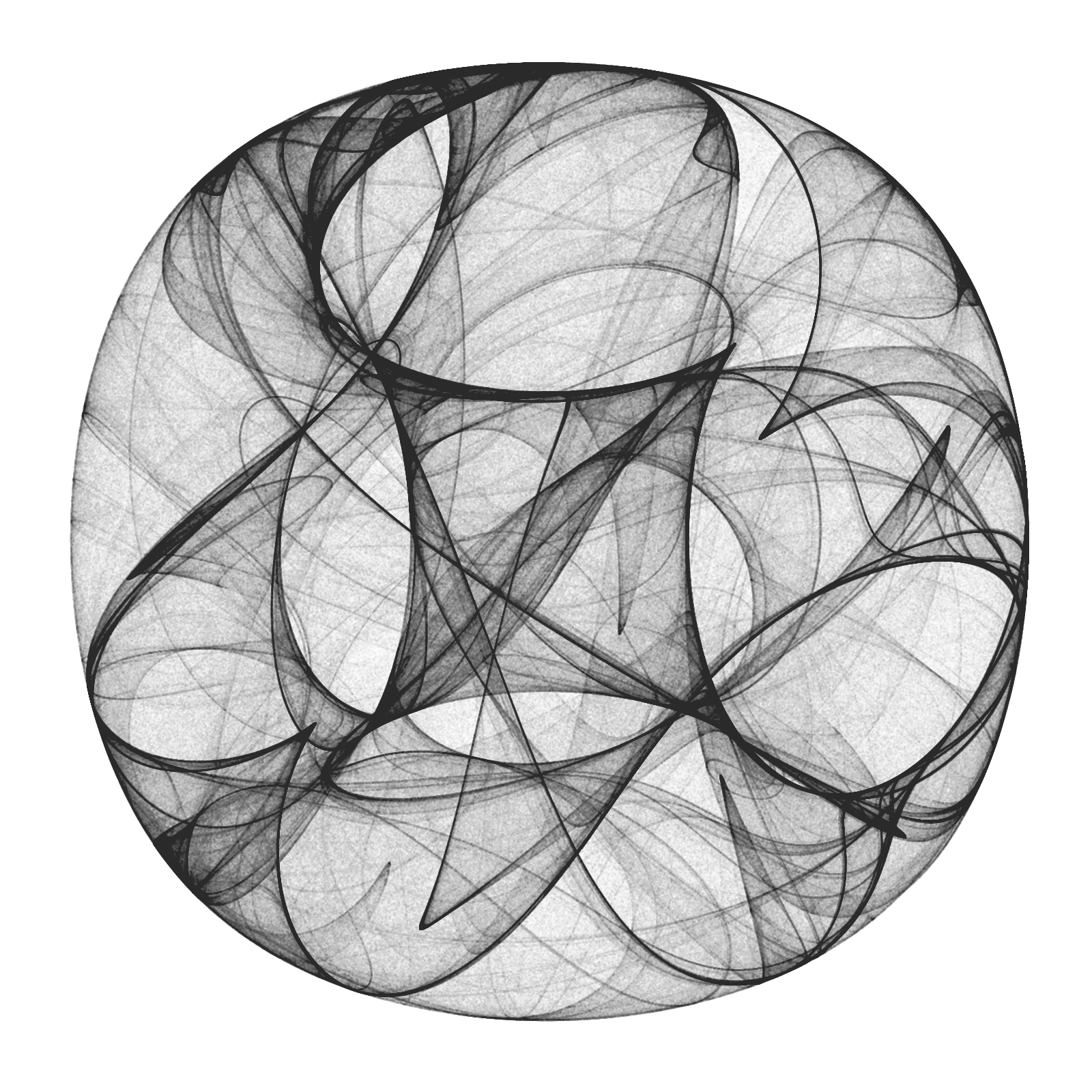Want to share your content on R-bloggers? click here if you have a blog, or here if you don't.

For me, mathematics cultivates a perpetual state of wonder about the nature of mind, the limits of thoughts, and our place in this vast cosmos (Clifford A. Pickover – The Math Book: From Pythagoras to the 57th Dimension, 250 Milestones in the History of Mathematics)

I am a big fan of Clifford Pickover and I find inspiration in his books very often. Thanks to him, I discovered the harmonograph and the Parrondo’s paradox, among many other mathematical treasures. Apart of being a great teacher, he also invented a family of strange attractors wearing his name. Clifford attractors are defined by these equations:$x_{n+1}\, =\, sin(a\, y_{n})\, +\, c\, cos(a\, x_{n}) \\ y_{n+1}\, =\, sin(b\, x_{n})\, +\, d\, cos(b\, y_{n}) \\$

There are infinite attractors, since a, b, c and d are parameters. Given four values (one for each parameter) and a starting point `(x0, y0)`, the previous equation defines the exact location of the point at step `n`, which is defined just by its location at `n-1`; an attractor can be thought as the trajectory described by a particle. This plot shows the evolution of a particle starting at `(x0, y0)=(0, 0)` with parameters `a=-1.24458046630025`, `b=-1.25191834103316`, `c=-1.81590817030519` and `d=-1.90866735205054` along 10 million of steps:Changing parameters is really entertaining. Drawings have a sandy appearance:From a technical point of view, the challenge is creating a data frame with all locations, since it must have 10 milion rows and must be populated sequentially. A very fast way to do it is using `Rcpp` package. To render the plot I use ggplot, which works quite well. Here you have the code to play with Clifford Attractors if you want:

```library(Rcpp)
library(ggplot2)
library(dplyr)

opt = theme(legend.position  = "none",
panel.background = element_rect(fill="white"),
axis.ticks       = element_blank(),
panel.grid       = element_blank(),
axis.title       = element_blank(),
axis.text        = element_blank())

cppFunction('DataFrame createTrajectory(int n, double x0, double y0,
double a, double b, double c, double d) {
// create the columns
NumericVector x(n);
NumericVector y(n);
x=x0;
y=y0;
for(int i = 1; i < n; ++i) {
x[i] = sin(a*y[i-1])+c*cos(a*x[i-1]);
y[i] = sin(b*x[i-1])+d*cos(b*y[i-1]);
}
// return a new data frame
return DataFrame::create(_["x"]= x, _["y"]= y);
}
')

a=-1.24458046630025
b=-1.25191834103316
c=-1.81590817030519
d=-1.90866735205054

df=createTrajectory(10000000, 0, 0, a, b, c, d)

png("Clifford.png", units="px", width=1600, height=1600, res=300)
ggplot(df, aes(x, y)) + geom_point(color="black", shape=46, alpha=.01) + opt
dev.off()
```